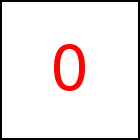# setModMat Library Method

Short Description: Set model translation matrix

Signature: x.setModMat (m)
Class: taccgl3DObject Class

Sets the model translation matrix to m. m must be a 4x4 matrix as explained in taccgl.Matrix.

The model translation matrix is used to transform the coordinates of the external 3D model into coordinates within the HTML element of the transition. Initially the matrix is the identity matrix. This means the point (0,0,0) given in coordinates of the external model refers to the top left corner of the HTML element. (1,0,0) refers to the top right corner and (0,1,0) to the bottom left corner. (0,0,1) refers to a point that is d pixels in front of the top left corner of the HTML element. d is the depth of the transition which initially is the height of the HTML element. It can be set with depth.

Note that in order to preserve the aspect ratio of the model, the underlying HTML element must have quadratic size, i.e. height = width.

By using a custom matrix, the model can be rotated, stretched, or moved as desired. In order to compute a suitable matrix the taccgl.Matrix functions are very helpful. It can also be useful to copy the model transition matrix of another transition, retrieved with the taccgl3DObject.getModMat.

The method taccgl3DObject.modFit is an alternative to set the model translation matrix.

WebGL™ is a trademark of the Khronos Group Inc.

Next Page:taccgl3DObject.getModMat - Get model translation matrix
Previous Page: taccgl3DObject.modFit - Align scene with HTML element

# taccgl3DObject Class

taccgl™ - Canvas Library